### Example 35.2 Principal Factor Analysis

This example uses the data presented in Example 35.1 and performs a principal factor analysis with squared multiple correlations for the prior communality estimates. Unlike Example 35.1, which analyzes the principal components (with default PRIORS=ONE), the current analysis is based on a common factor model. To use a common factor model, you specify PRIORS=SMC in the PROC FACTOR statement, as shown in the following:

```
ods graphics on;

proc factor data=SocioEconomics
priors=smc msa residual
rotate=promax reorder
outstat=fact_all
run;
```
```ods graphics off;
```

In the PROC FACTOR statement, you include several other options to help you analyze the results. To help determine whether the common factor model is appropriate, you request the Kaiser’s measure of sampling adequacy with the MSA option. You specify the RESIDUALS option to compute the residual correlations and partial correlations.

The ROTATE= and REORDER options are specified to enhance factor interpretability. The ROTATE=PROMAX option produces an orthogonal varimax prerotation (default) followed by an oblique Procrustes rotation, and the REORDER option reorders the variables according to their largest factor loadings. An OUTSTAT= data set is created by PROC FACTOR and displayed in Output 35.2.15.

PROC FACTOR can produce high-quality graphs that are very useful for interpreting the factor solutions. To request these graphs, ODS Graphics must be enabled. All ODS graphs in PROC FACTOR are requested with the PLOTS= option. In this example, you request a scree plot (SCREE) and loading plots for the factor matrix during the following three stages: initial unrotated solution (INITLOADINGS), prerotated (varimax) solution (PRELOADINGS), and promax-rotated solution (LOADINGS). The scree plot helps you determine the number of factors, and the loading plots help you visualize the patterns of factor loadings during various stages of analyses.

#### Principal Factor Analysis: Kaiser’s MSA and Factor Extraction Results

Output 35.2.1 displays the results of the partial correlations and Kaiser’s measure of sampling adequacy.

Output 35.2.1: Principal Factor Analysis: Partial Correlations and Kaiser’s MSA

Partial Correlations Controlling all other Variables
Population School Employment Services HouseValue
Population 1.00000 -0.54465 0.97083 0.09612 0.15871
School -0.54465 1.00000 0.54373 0.04996 0.64717
Employment 0.97083 0.54373 1.00000 0.06689 -0.25572
Services 0.09612 0.04996 0.06689 1.00000 0.59415
HouseValue 0.15871 0.64717 -0.25572 0.59415 1.00000

Kaiser's Measure of Sampling Adequacy: Overall MSA = 0.57536759
Population School Employment Services HouseValue
0.47207897 0.55158839 0.48851137 0.80664365 0.61281377

If the data are appropriate for the common factor model, the partial correlations (controlling all other variables) should be small compared to the original correlations. For example, the partial correlation between the variables `School` and `HouseValue` is 0.65, slightly less than the original correlation of 0.86 (see Output 35.1.3). The partial correlation between `Population` and `School` is –0.54, which is much larger in absolute value than the original correlation; this is an indication of trouble. Kaiser’s MSA is a summary, for each variable and for all variables together, of how much smaller the partial correlations are than the original correlations. Values of 0.8 or 0.9 are considered good, while MSAs below 0.5 are unacceptable. The variables `Population`, `School`, and `Employment` have very poor MSAs. Only the `Services` variable has a good MSA. The overall MSA of 0.58 is sufficiently poor that additional variables should be included in the analysis to better define the common factors. A commonly used rule is that there should be at least three variables per factor. In the following analysis, you determine that there are two common factors in these data. Therefore, more variables are needed for a reliable analysis.

Output 35.2.2 displays the results of the principal factor extraction.

Output 35.2.2: Principal Factor Analysis: Factor Extraction

Prior Communality Estimates: SMC
Population School Employment Services HouseValue
0.96859160 0.82228514 0.96918082 0.78572440 0.84701921

Eigenvalues of the Reduced Correlation Matrix: Total = 4.39280116 Average = 0.87856023
Eigenvalue Difference Proportion Cumulative
1 2.73430084 1.01823217 0.6225 0.6225
2 1.71606867 1.67650586 0.3907 1.0131
3 0.03956281 0.06408626 0.0090 1.0221
4 -.02452345 0.04808427 -0.0056 1.0165
5 -.07260772   -0.0165 1.0000

The square multiple correlations are shown as prior communality estimates in Output 35.2.2. The PRIORS=SMC option basically replaces the diagonal of the original observed correlation matrix by these square multiple correlations. Because the square multiple correlations are usually less than one, the resulting correlation matrix for factoring is called the reduced correlation matrix. In the current example, the SMCs are all fairly large; hence, you expect the results of the principal factor analysis to be similar to those in the principal component analysis.

The first two largest positive eigenvalues of the reduced correlation matrix account for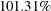of the common variance. This is possible because the reduced correlation matrix, in general, is not necessarily positive definite, and negative eigenvalues for the matrix are possible. A pattern like this suggests that you might not need more than two common factors. The scree and variance explained plots of Output 35.2.3 clearly support the conclusion that two common factors are present. Showing in the left panel of Output 35.2.3 is the scree plot of the eigenvalues of the reduced correlation matrix. A sharp bend occurs at the third eigenvalue, reinforcing the conclusion that two common factors are present. These cumulative proportions of common variance explained by factors are plotted in the right panel of Output 35.2.3, which shows that the curve essentially flattens out after the second factor.

Output 35.2.3: Scree and Variance Explained Plots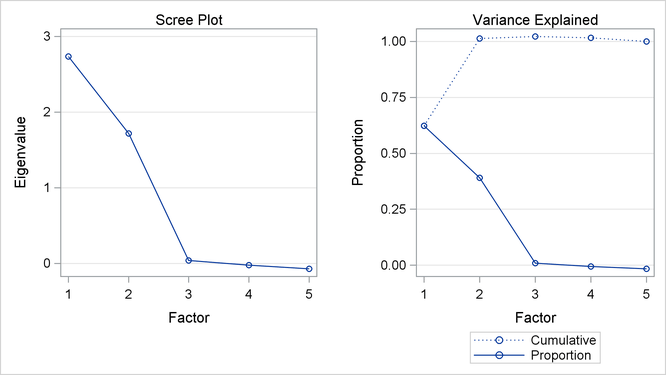#### Principal Factor Analysis: Initial Factor Solution

For the current analysis, PROC FACTOR retains two factors by certain default criteria. This decision agrees with the conclusion drawn by inspecting the scree plot. The principal factor pattern with the two factors is displayed in Output 35.2.4. This factor pattern is similar to the principal component pattern seen in Output 35.1.5 of Example 35.1. For example, the variable `Services` has the largest loading on the first factor, and the `Population` variable has the smallest. The variables `Population` and `Employment` have large positive loadings on the second factor, and the `HouseValue` and `School` variables have large negative loadings.

Output 35.2.4: Initial Factor Pattern Matrix and Communalities

Factor Pattern
Factor1 Factor2
Services 0.87899 -0.15847
HouseValue 0.74215 -0.57806
Employment 0.71447 0.67936
School 0.71370 -0.55515
Population 0.62533 0.76621

Variance Explained by Each
Factor
Factor1 Factor2
2.7343008 1.7160687

Final Communality Estimates: Total = 4.450370
Population School Employment Services HouseValue
0.97811334 0.81756387 0.97199928 0.79774304 0.88494998

Comparing the current factor loading matrix in Output 35.2.4 with that in Output 35.1.5 in Example 35.1, you notice that the variables are arranged differently in the two output tables. This is due to the use of the REORDER option in the current analysis. The advantage of using this option might not be very obvious in Output 35.2.4, but you can see its value when looking at the rotated solutions, as shown in Output 35.2.7 and Output 35.2.11.

The final communality estimates are all fairly close to the priors (shown in Output 35.2.2). Only the communality for the variable `HouseValue` increased appreciably, from 0.847 to 0.885. Therefore, you are sure that all the common variance is accounted for.

Output 35.2.5 shows that the residual correlations (off-diagonal elements) are low, the largest being 0.03. The partial correlations are not quite as impressive, since the uniqueness values are also rather small. These results indicate that the squared multiple correlations are good but not quite optimal communality estimates.

Output 35.2.5: Residual and Partial Correlations

Residual Correlations With Uniqueness on the Diagonal
Population School Employment Services HouseValue
Population 0.02189 -0.01118 0.00514 0.01063 0.00124
School -0.01118 0.18244 0.02151 -0.02390 0.01248
Employment 0.00514 0.02151 0.02800 -0.00565 -0.01561
Services 0.01063 -0.02390 -0.00565 0.20226 0.03370
HouseValue 0.00124 0.01248 -0.01561 0.03370 0.11505

Root Mean Square Off-Diagonal Residuals: Overall = 0.01693282
Population School Employment Services HouseValue
0.00815307 0.01813027 0.01382764 0.02151737 0.01960158

Partial Correlations Controlling Factors
Population School Employment Services HouseValue
Population 1.00000 -0.17693 0.20752 0.15975 0.02471
School -0.17693 1.00000 0.30097 -0.12443 0.08614
Employment 0.20752 0.30097 1.00000 -0.07504 -0.27509
Services 0.15975 -0.12443 -0.07504 1.00000 0.22093
HouseValue 0.02471 0.08614 -0.27509 0.22093 1.00000

Root Mean Square Off-Diagonal Partials: Overall = 0.18550132
Population School Employment Services HouseValue
0.15850824 0.19025867 0.23181838 0.15447043 0.18201538

As displayed in Output 35.2.6, the unrotated factor pattern reveals two tight clusters of variables, with the variables `HouseValue` and `School` at the negative end of `Factor2` axis and the variables `Employment` and `Population` at the positive end. The `Services` variable is in between but closer to the `HouseValue` and `School` variables. A good rotation would place the axes so that most variables would have zero loadings on most factors. As a result, the axes would appear as though they are put through the variable clusters.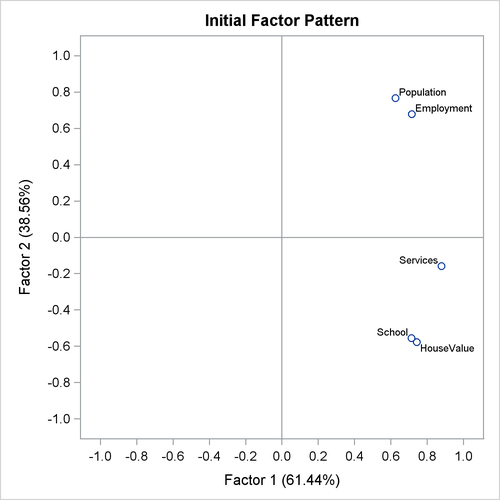#### Principal Factor Analysis: Varimax Prerotation

In Output 35.2.7, the results of the varimax prerotation are shown. To yield the varimax-rotated factor loading (pattern), the initial factor loading matrix is postmultiplied by an orthogonal transformation matrix. This orthogonal transformation matrix is shown in Output 35.2.7, followed by the varimax-rotated factor pattern. This rotation or transformation leads to small loadings of `Population` and `Employment` on the first factor and small loadings of `HouseValue` and `School` on the second factor. `Services` appears to have a larger loading on the first factor than it has on the second factor, although both loadings are substantial. Hence, `Services` appears to be factorially complex.

With the REORDER option in effect, you can see the variable clusters clearly in the factor pattern. The first factor is associated more with the first three variables (first three rows of variables): `HouseValue`, `School`, and `Services`. The second factor is associated more with the last two variables (last two rows of variables): `Population` and `Employment`.

For orthogonal factor solutions such as the current varimax-rotated solution, you can also interpret the values in the factor loading (pattern) matrix as correlations. For example, `HouseValue` and `Factor 1` have a high correlation at 0.94, while `Population` and `Factor 1` have a low correlation at 0.02.

Output 35.2.7: Varimax Rotation: Transform Matrix and Rotated Pattern

Orthogonal Transformation Matrix
1 2
1 0.78895 0.61446
2 -0.61446 0.78895

Rotated Factor Pattern
Factor1 Factor2
HouseValue 0.94072 -0.00004
School 0.90419 0.00055
Services 0.79085 0.41509
Population 0.02255 0.98874
Employment 0.14625 0.97499

Variance Explained by Each
Factor
Factor1 Factor2
2.3498567 2.1005128

Final Communality Estimates: Total = 4.450370
Population School Employment Services HouseValue
0.97811334 0.81756387 0.97199928 0.79774304 0.88494998

The variance explained by the factors are more evenly distributed in the varimax-rotated solution, as compared with that of the unrotated solution. Indeed, this is a typical fact for any kinds of factor rotation. In the current example, before the varimax rotation the two factors explain 2.73 and 1.72, respectively, of the common variance (see Output 35.2.4). After the varimax rotation the two rotated factors explain 2.35 and 2.10, respectively, of the common variance. However, the total variance accounted for by the factors remains unchanged after the varimax rotation. This invariance property is also observed for the communalities of the variables after the rotation, as evidenced by comparing the current communality estimates in Output 35.2.7 with those in Output 35.2.4.

Output 35.2.8 shows the graphical plot of the varimax-rotated factor loadings. Clearly, `HouseValue` and `School` cluster together on the `Factor 1` axis, while `Population` and `Employment` cluster together on the `Factor 2` axis. `Service` is closer to the cluster of `HouseValue` and `School`.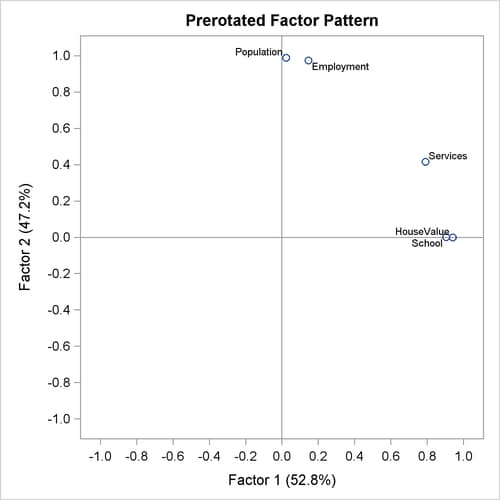An alternative to the scatter plot of factor loadings is the so-called vector plot of loadings, which is shown in Output 35.2.9. The vector plot is requested with the suboption VECTOR in the PLOTS= option. That is:

```plots=preloadings(vector)
```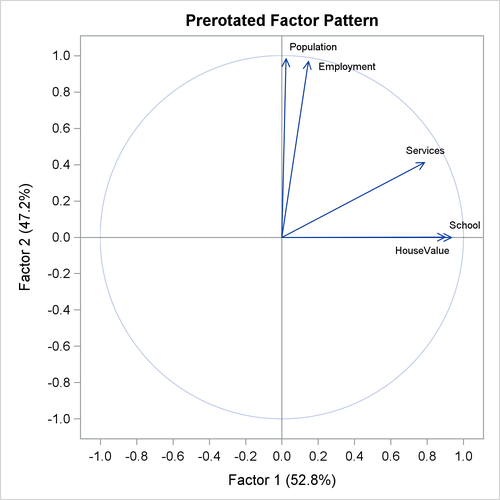#### Principal Factor Analysis: Oblique Promax Rotation

For some researchers, the varimax-rotated factor solution in the preceding section might be good enough to provide them useful and interpretable results. For others who believe that common factors are seldom orthogonal, an obliquely rotated factor solution might be more desirable, or at least should be attempted.

PROC FACTOR provides a very large class of oblique factor rotations. The current example shows a particular one—namely, the promax rotation as requested by the ROTATE=PROMAX option.

The results of the promax rotation are shown in Output 35.2.10 and Output 35.2.11. The corresponding plot of factor loadings is shown in Output 35.2.12.

Output 35.2.10: Promax Rotation: Procrustean Target and Transformation

Target Matrix for Procrustean Transformation
Factor1 Factor2
HouseValue 1.00000 -0.00000
School 1.00000 0.00000
Services 0.69421 0.10045
Population 0.00001 1.00000
Employment 0.00326 0.96793

Procrustean Transformation Matrix
1 2
1 1.04116598 -0.0986534
2 -0.1057226 0.96303019

Normalized Oblique Transformation
Matrix
1 2
1 0.73803 0.54202
2 -0.70555 0.86528

Output 35.2.10 shows the Procrustean target, to which the varimax factor pattern is rotated, followed by the display of the Procrustean transformation matrix. This is the matrix that transforms the varimax factor pattern so that the rotated pattern is as close as possible to the Procrustean target. However, because the variances of factors have to be fixed at 1 during the oblique transformation, a normalized version of the Procrustean transformation matrix is the one that is actually used in the transformation. This normalized transformation matrix is shown at the bottom of Output 35.2.10. Using this transformation matrix leads to the promax-rotated factor solution, as shown in Output 35.2.11.

Output 35.2.11: Promax Rotation: Factor Correlations and Factor Pattern

Inter-Factor Correlations
Factor1 Factor2
Factor1 1.00000 0.20188
Factor2 0.20188 1.00000

Rotated Factor Pattern (Standardized Regression Coefficients)
Factor1 Factor2
HouseValue 0.95558485 -0.0979201
School 0.91842142 -0.0935214
Services 0.76053238 0.33931804
Population -0.0790832 1.00192402
Employment 0.04799 0.97509085

After the promax rotation, the factors are no longer uncorrelated. As shown in Output 35.2.11, the correlation of the two factors is now 0.20. In the (initial) unrotated and the varimax solutions, the two factors are not correlated.

In addition to allowing the factors to be correlated, in an oblique factor solution you seek a pattern of factor loadings that is more differentiated (referred to as the simple structures in the literature). The more differentiated the loadings, the easier the interpretation of the factors.

For example, factor loadings of `Services` and `Population` on `Factor 2` are 0.415 and 0.989, respectively, in the (orthogonal) varimax-rotated factor pattern (see Output 35.2.7). With the (oblique) promax rotation (see Output 35.2.11), these two loadings become even more differentiated with values 0.339 and 1.002, respectively. Overall, however, the factor patterns before and after the promax rotation do not seem to differ too much. This fact is confirmed by comparing the graphical plots of factor loadings. The plots in Output 35.2.12 (promax-rotated factor loadings) and Output 35.2.8 (varimax-rotated factor loadings) show very similar patterns.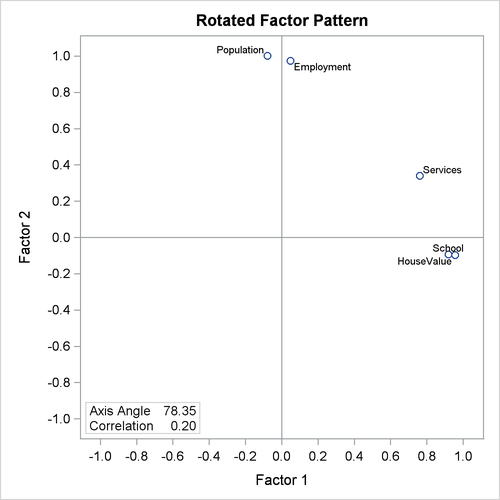Unlike the orthogonal factor solutions where you can interpret the factor loadings as correlations between variables and factors, in oblique factor solutions such as the promax solution, you have to turn to the factor structure matrix for examining the correlations between variables and factors. Output 35.2.13 shows the factor structures of the promax-rotated solution.

Output 35.2.13: Promax Rotation: Factor Structures and Final Communalities

Factor Structure (Correlations)
Factor1 Factor2
HouseValue 0.93582 0.09500
School 0.89954 0.09189
Services 0.82903 0.49286
Population 0.12319 0.98596
Employment 0.24484 0.98478

Variance Explained by Each
Factor Ignoring Other Factors
Factor1 Factor2
2.4473495 2.2022803

Final Communality Estimates: Total = 4.450370
Population School Employment Services HouseValue
0.97811334 0.81756387 0.97199928 0.79774304 0.88494998

Basically, the factor structure matrix shown in Output 35.2.13 reflects a similar pattern to the factor pattern matrix shown in Output 35.2.11. The critical difference is that you can have the correlation interpretation only by using the factor structure matrix. For example, in the factor structure matrix shown in Output 35.2.13, the correlation between `Population` and `Factor 2` is 0.986. The corresponding value shown in the factor pattern matrix in Output 35.2.11 is 1.002, which certainly cannot be interpreted as a correlation coefficient.

Common variance explained by the promax-rotated factors are 2.447 and 2.202, respectively, for the two factors. Unlike the orthogonal factor solutions (for example, the prerotated varimax solution), variance explained by these promax-rotated factors do not sum up to the total communality estimate 4.45. In oblique factor solutions, variance explained by oblique factors cannot be partitioned for the factors. Variance explained by a common factor is computed while ignoring the contributions from the other factors.

However, the communalities for the variables, as shown in the bottom of Output 35.2.13, do not change from rotation to rotation. They are still the same set of communalities in the initial, varimax-rotated, and promax-rotated solutions. This is a basic fact about factor rotations: they only redistribute the variance explained by the factors; the total variance explained by the factors for any variable (that is, the communality of the variable) remains unchanged.

In the literature of exploratory factor analysis, reference axes had been an important tool in factor rotation. Nowadays, rotations are seldom done through the uses of the reference axes. Despite that, results about reference axes do provide additional information for interpreting factor analysis results. For the current example of the promax rotation, PROC FACTOR shows the relevant results about the reference axes in Output 35.2.14.

Output 35.2.14: Promax Rotation: Reference Axis Correlations and Reference Structures

Reference Axis Correlations
Factor1 Factor2
Factor1 1.00000 -0.20188
Factor2 -0.20188 1.00000

Reference Structure (Semipartial Correlations)
Factor1 Factor2
HouseValue 0.93591 -0.09590
School 0.89951 -0.09160
Services 0.74487 0.33233
Population -0.07745 0.98129
Employment 0.04700 0.95501

Variance Explained by Each
Factor Eliminating Other
Factors
Factor1 Factor2
2.2480892 2.0030200

To explain the results in the reference-axis system, some geometric interpretations of the factor axes are needed. Consider a single factor in a system of n common factors in an oblique factor solution. Taking away the factor under consideration, the remaining n – 1 factors span a hyperplane in the factor space of n – 1 dimensions. The vector that is orthogonal to this hyperplane is the reference axis (reference vector) of the factor under consideration. Using the same definition for the remaining factors, you have n reference vectors for n factors.

A factor in an oblique factor solution can be considered as the sum of two independent components: its associated reference vector and a component that is overlapped with all other factors. In other words, the reference vector of a factor is a unique part of the factor that is not predictable from all other factors. Thus, the loadings on a reference vector are the unique effects of the corresponding factor, partialling out the effects from all other factors. The variances explained by a reference vector are the unique variances explained by the corresponding factor, partialling out the variances explained by all other factors.

Output 35.2.14 shows the reference axis correlations. The correlation between the reference vectors is –0.20. Next, Output 35.2.14 shows the loadings on the reference vectors in the table entitled Reference Structure (Semipartial Correlations). As explained previously, loadings on a reference vector are also the unique effects of the corresponding factor, partialling out the effects from the all other factors. For example, the unique effect of `Factor 1` on `HouseValue` is 0.936. Another important property of the reference vector system is that loadings on a reference vector are also correlations between the variables and the corresponding factor, partialling out the correlations between the variables and other factors. This means that the loading 0.936 in the reference structure table is the unique correlation between `HouseValue` and `Factor 1`, partialling out the correlation between `HouseValue` with `Factor 2`. Hence, as suggested by the title of table, all loadings reported in the Reference Structure (Semipartial Correlations) can be interpreted as semipartial correlations between variables and factors.

The last table shown in Output 35.2.14 are the variances explained by the reference vectors. As explained previously, these are also unique variances explained by the factors, partialling out the variances explained by all other factors (or eliminating all other factors, as suggested by the title of the table). In the current example, `Factor 1` explains 2.248 of the variable variances, partialling out all variable variances explained by `Factor 2`.

Notice that factor pattern (shown in Output 35.2.11), factor structures (correlations, shown in Output 35.2.13), and reference structures (semipartial correlations, shown in Output 35.2.14) give you different information about the oblique factor solutions such as the promax-rotated solution. However, for orthogonal factor solutions such as the varimax-rotated solution, factor structures and reference structures are all the same as the factor pattern.

#### Principal Factor Analysis: Factor Rotations with Factor Pattern Input

The promax rotation is one of the many rotations that PROC FACTOR provides. You can specify many different rotation algorithms by using the ROTATE= options. In this section, you explore different rotated factor solutions from the initial principal factor solution. Specifically, you want to examine the factor patterns yielded by the quartimax transformation (an orthogonal transformation) and the Harris-Kaiser (an oblique transformation), respectively.

Rather than analyzing the entire problem again with new rotations, you can simply use the OUTSTAT= data set from the preceding factor analysis results.

First, the OUTSTAT= data set is printed using the following statements:

```
proc print data=fact_all;
run;

```

The output data set is displayed in Output 35.2.15.

Output 35.2.15: Output Data Set

 Factor Output Data Set

Obs _TYPE_ _NAME_ Population School Employment Services HouseValue
1 MEAN   6241.67 11.4417 2333.33 120.833 17000.00
2 STD   3439.99 1.7865 1241.21 114.928 6367.53
3 N   12.00 12.0000 12.00 12.000 12.00
4 CORR Population 1.00 0.0098 0.97 0.439 0.02
5 CORR School 0.01 1.0000 0.15 0.691 0.86
6 CORR Employment 0.97 0.1543 1.00 0.515 0.12
7 CORR Services 0.44 0.6914 0.51 1.000 0.78
8 CORR HouseValue 0.02 0.8631 0.12 0.778 1.00
9 COMMUNAL   0.98 0.8176 0.97 0.798 0.88
10 PRIORS   0.97 0.8223 0.97 0.786 0.85
11 EIGENVAL   2.73 1.7161 0.04 -0.025 -0.07
12 UNROTATE Factor1 0.63 0.7137 0.71 0.879 0.74
13 UNROTATE Factor2 0.77 -0.5552 0.68 -0.158 -0.58
14 RESIDUAL Population 0.02 -0.0112 0.01 0.011 0.00
15 RESIDUAL School -0.01 0.1824 0.02 -0.024 0.01
16 RESIDUAL Employment 0.01 0.0215 0.03 -0.006 -0.02
17 RESIDUAL Services 0.01 -0.0239 -0.01 0.202 0.03
18 RESIDUAL HouseValue 0.00 0.0125 -0.02 0.034 0.12
19 PRETRANS Factor1 0.79 -0.6145 . . .
20 PRETRANS Factor2 0.61 0.7889 . . .
21 PREROTAT Factor1 0.02 0.9042 0.15 0.791 0.94
22 PREROTAT Factor2 0.99 0.0006 0.97 0.415 -0.00
23 TRANSFOR Factor1 0.74 -0.7055 . . .
24 TRANSFOR Factor2 0.54 0.8653 . . .
25 FCORR Factor1 1.00 0.2019 . . .
26 FCORR Factor2 0.20 1.0000 . . .
27 PATTERN Factor1 -0.08 0.9184 0.05 0.761 0.96
28 PATTERN Factor2 1.00 -0.0935 0.98 0.339 -0.10
29 RCORR Factor1 1.00 -0.2019 . . .
30 RCORR Factor2 -0.20 1.0000 . . .
31 REFERENC Factor1 -0.08 0.8995 0.05 0.745 0.94
32 REFERENC Factor2 0.98 -0.0916 0.96 0.332 -0.10
33 STRUCTUR Factor1 0.12 0.8995 0.24 0.829 0.94
34 STRUCTUR Factor2 0.99 0.0919 0.98 0.493 0.09

Various results from the previous factor analysis are saved in this data set, including the initial unrotated solution (its factor pattern is saved in observations with `_TYPE_`=UNROTATE), the prerotated varimax solution (its factor pattern is saved in observations with `_TYPE_`=PREROTAT), and the oblique promax solution (its factor pattern is saved in observations with `_TYPE_`=PATTERN).

When PROC FACTOR reads in an input data set with TYPE=FACTOR, the observations with `_TYPE_`=PATTERN are treated as the initial factor pattern to be rotated by PROC FACTOR. Hence, it is important that you provide the correct initial factor pattern for PROC FACTOR to read in.

In the current example, you need to provide the unrotated solution from the preceding analysis as the input factor pattern. The following statements create a TYPE=FACTOR data set `fact2` from the preceding OUTSTAT= data set `fact_all`:

```data fact2(type=factor);
set fact_all;
if _TYPE_ in('PATTERN' 'FCORR') then delete;
if _TYPE_='UNROTATE' then _TYPE_='PATTERN';
run;
```

In these statements, you delete observations with `_TYPE_`=PATTERN or `_TYPE_`=FCORR, which are for the promax-rotated factor solution, and change observations with `_TYPE_`=UNROTATE to `_TYPE_`=PATTERN in the new data set `fact2`. In this way, the initial orthogonal factor pattern matrix is saved in the observations with `_TYPE_`=PATTERN.

You use this new data set and rotate the initial solution to another oblique solution with the ROTATE=QUARTIMAX option, as shown in the following statements:

```proc factor data=fact2 rotate=quartimax reorder;
run;
```

As shown in Output 35.2.16, the input data set is of the `FACTOR` type for the new rotation.

Output 35.2.16: Quartimax Rotation With Input Factor Pattern

 Quartimax Rotation From a TYPE=FACTOR Data Set

The FACTOR Procedure

Input Data Type FACTOR 12 12

The quartimax-rotated factor pattern is displayed in Output 35.2.17.

Output 35.2.17: Quartimax-Rotated Factor Pattern

Orthogonal Transformation Matrix
1 2
1 0.80138 0.59815
2 -0.59815 0.80138

Rotated Factor Pattern
Factor1 Factor2
HouseValue 0.94052 -0.01933
School 0.90401 -0.01799
Services 0.79920 0.39878
Population 0.04282 0.98807
Employment 0.16621 0.97179

Variance Explained by Each
Factor
Factor1 Factor2
2.3699941 2.0803754

The quartimax rotation produces an orthogonal transformation matrix shown at the top of Output 35.2.17. After the transformation, the factor pattern is shown next. Compared with the varimax-rotated factor pattern (see Output 35.2.7), the quartimax-rotated factor pattern shows some differences. The loadings of `HouseValue` and `School` on `Factor 1` drop only slightly in the quartimax factor pattern, while the loadings of `Services`, `Population`, and `Employment` on `Factor 1` gain relatively larger amounts. The total variance explained by `Factor 1` in the varimax-rotated solution (see Output 35.2.7) is 2.350, while it is 2.370 after the quartimax-rotation. In other words, more variable variances are explained by the first factor in the quartimax factor pattern than in the varimax factor pattern. Although not very strongly demonstrated in the current example, this illustrates a well-known property about the quartimax rotation: it tends to produce a general factor for all variables.

Another oblique rotation is now explored. The Harris-Kaiser transformation weighted by the Cureton-Mulaik technique is applied to the initial factor pattern. To achieve this, you use the ROTATE=HK and NORM=WEIGHT options in the following PROC FACTOR statement:

```ods graphics on;
```
```proc factor data=fact2 rotate=hk norm=weight reorder
run;

ods graphics off;

```

Output 35.2.18 shows the variable weights in the rotation.

Output 35.2.18: Harris-Kaiser Rotation: Weights

Variable Weights for Rotation
Population School Employment Services HouseValue
0.95982747 0.93945424 0.99746396 0.12194766 0.94007263

While all other variables have weights at least as large as 0.93, the weight for `Services` is only 0.12. This means that due to its small weight, `Services` is not as important as the other variables for determining the rotation (transformation). This makes sense when you look at the initial unrotated factor pattern plot in Output 35.2.6. In the plot, there are two main clusters of variables, and `Services` does not seem to fall into either of the clusters. In order to yield a Harris-Kaiser rotation (transformation) that would gear towards to two clusters, the Cureton-Mulaik weighting essentially downweights the contribution from `Services` in the factor rotation.

The results of the Harris-Kaiser factor solution are displayed in Output 35.2.19, with a graphical plot of rotated loadings displayed in Output 35.2.20.

Output 35.2.19: Harris-Kaiser Rotation: Factor Correlations and Factor Pattern

Inter-Factor Correlations
Factor1 Factor2
Factor1 1.00000 0.08358
Factor2 0.08358 1.00000

Rotated Factor Pattern (Standardized Regression Coefficients)
Factor1 Factor2
HouseValue 0.94048 0.00279
School 0.90391 0.00327
Services 0.75459 0.41892
Population -0.06335 0.99227
Employment 0.06152 0.97885

Because the Harris-Kaiser produces an oblique factor solution, you compare the current results with that of the promax (see Output 35.2.11), which also produces an oblique factor solution. The correlation between the factors in the Harris-Kaiser solution is 0.084; this value is much smaller than the same correlation in the promax solution, which is 0.201. However, the Harris-Kaiser rotated factor pattern shown in Output 35.2.19 is more or less the same as that of the promax-rotated factor pattern shown in Output 35.2.11. Which solution would you consider to be more reasonable or interpretable?

From the statistical point of view, the Harris-Kaiser and promax factor solutions are equivalent. They explain the observed variable relationships equally well. From the simplicity point of view, however, you might prefer to interpret the Harris-Kaiser solution because the factor correlation is smaller. In other words, the factors in the Harris-Kaiser solution do not overlap that much conceptually; hence they should be more distinctive to interpret. However, in practice simplicity in factor correlations might not the only principle to consider. Researchers might actually expect to have some factors to be highly correlated based on theoretical or substantive grounds.

Although the Harris-Kaiser and the promax factor patterns are very similar, the graphical plots of the loadings from the two solutions paint slightly different pictures. The plot of the promax-rotated loadings is shown in Output 35.2.12, while the plot of the loadings for the current Harris-Kaiser solution is shown in Output 35.2.20.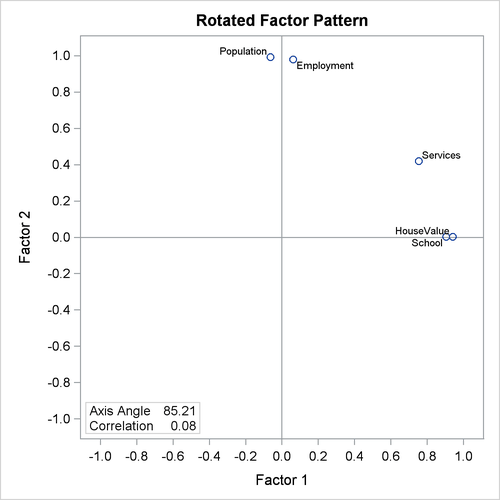The two factor axes in the Harris-Kaiser rotated pattern (Output 35.2.20) clearly cut through the centers of the two variable clusters, while the `Factor 1` axis in the promax solution lies above a variable cluster (Output 35.2.12). The reason for this subtle difference is that in the Harris-Kaiser rotation, the `Services` is a loner that has been downweighted by the Cureton-Mulaik technique (see its relatively small weight in Output 35.2.18). As a result, the rotated axes are basically determined by the two variable clusters in the Harris-Kaiser rotation.